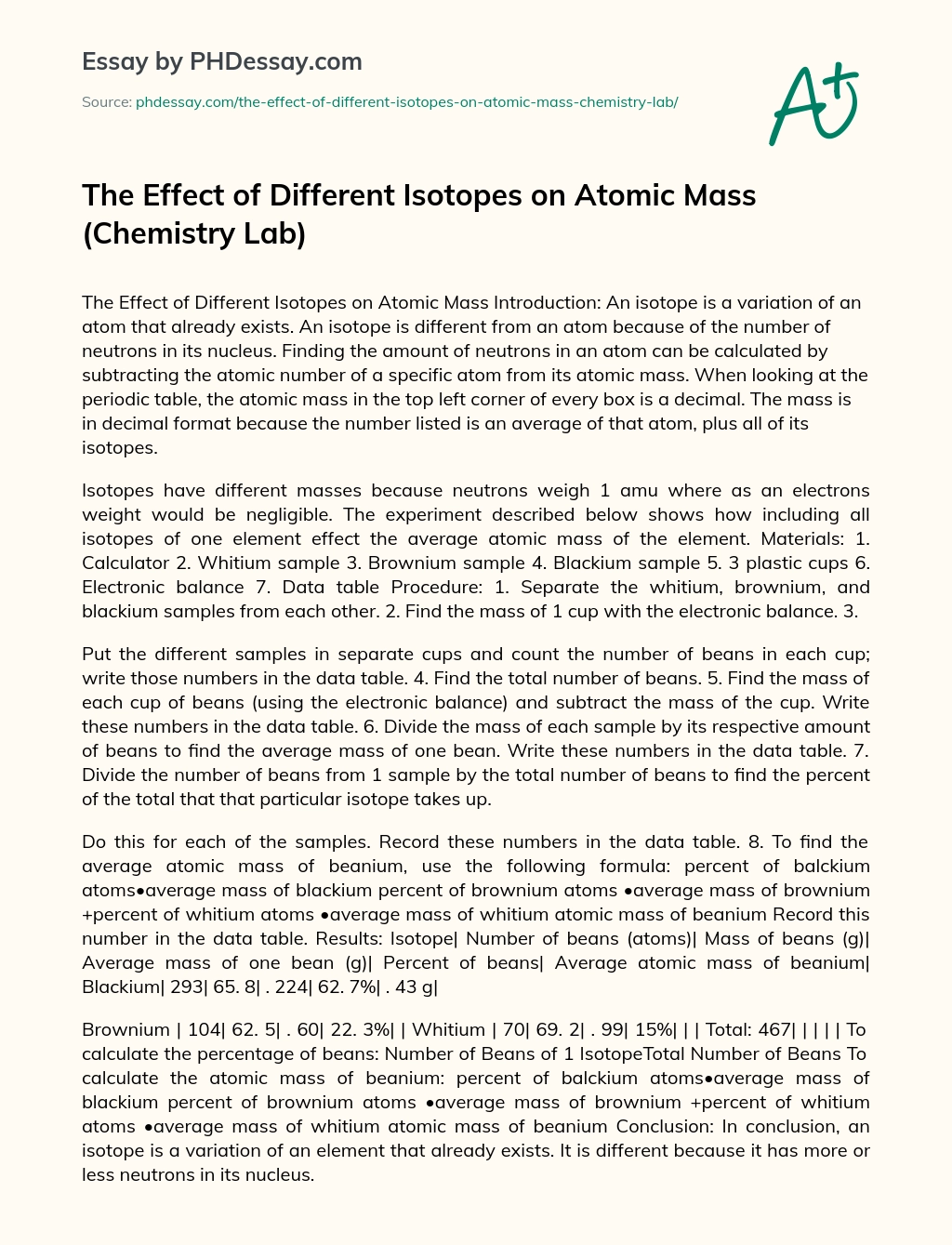Last Updated 20 Apr 2022

# The Effect of Different Isotopes on Atomic Mass (Chemistry Lab)

Category Chemistry, Nature, Physics
Words 726 (3 pages)
Views 542

## Introduction

An isotope is a variation of an atom that already exists. An isotope is different from an atom because of the number of neutrons in its nucleus. Finding the amount of neutrons in an atom can be calculated by subtracting the atomic number of a specific atom from its atomic mass. When looking at the periodic table, the atomic mass in the top left corner of every box is a decimal. The mass is in decimal format because the number listed is an average of that atom, plus all of its isotopes.

Isotopes have different masses because neutrons weigh 1 amu where as an electrons weight would be negligible. The experiment described below shows how including all isotopes of one element effect the average atomic mass of the element. Materials:

Order custom essay The Effect of Different Isotopes on Atomic Mass (Chemistry Lab) with free plagiarism report

GET ORIGINAL PAPER
1.  Calculator
2. Whitium sample
3.  Brownium sample
4.  Blackium sample
5. 3 plastic cups
6. Electronic balance
7. Data table

Procedure:

1. Separate the whitium, brownium, and blackium samples from each other.
2. Find the mass of 1 cup with the electronic balance.
3. Put the different samples in separate cups and count the number of beans in each cup; write those numbers in the data table.
4.  Find the total number of beans.
5. Find the mass of each cup of beans (using the electronic balance) and subtract the mass of the cup. Write these numbers in the data table.
6.  Divide the mass of each sample by its respective amount of beans to find the average mass of one bean. Write these numbers in the data table.
7. Divide the number of beans from 1 sample by the total number of beans to find the percent of the total that that particular isotope takes up. Do this for each of the samples. Record these numbers in the data table.
8. To find the average atomic mass of beanium, use the following formula: percent of balckium atoms•average mass of blackium percent of brownium atoms •average mass of brownium +percent of whitium atoms •average mass of whitium atomic mass of beanium Record this number in the data table.

Results: Isotope| Number of beans (atoms)| Mass of beans (g)| Average mass of one bean (g)| Percent of beans| Average atomic mass of beanium| Blackium| 293| 65. 8| . 224| 62. 7%| . 43 g|

Brownium | 104| 62. 5| . 60| 22. 3%| | Whitium | 70| 69. 2| . 99| 15%| | | Total: 467| | | | | To calculate the percentage of beans: Number of Beans of 1 IsotopeTotal Number of Beans To calculate the atomic mass of beanium: percent of balckium atoms•average mass of blackium percent of brownium atoms •average mass of brownium +percent of whitium atoms •average mass of whitium atomic mass of beanium Conclusion: In conclusion, an isotope is a variation of an element that already exists. It is different because it has more or less neutrons in its nucleus.

Depending on how many isotopes one element has, the average atomic mass will change. When calculating the average atomic mass, you must include all of the isotopes which have more or less neutrons than the original element. Since neutrons have a mass of 1amu, the isotopes masses will vary, thus affecting the average atomic mass of an element. When performing this experiment, the mass of the beans were measured while the number of beans, average mass and percent of beans had to be calculated. The average mass of he beans, or isotopes, was a decimal because the weight of the beans in one sample divided by the number of beans of the same sample was not an even number. This lab simulates the various isotopes of an element because all of the beans were in the same ‘family’; however, they all looked different and had different masses. This is an example of how real elements have isotopes that may not look alike or have the same mass, but they’re still a part of that one element. As this experiment may have gotten the results shown above, when performing this experiment a second time, the results may vary.

This is because not every bean is identical. If larger samples are used then the difference may be smaller because the larger the sample you have to work with, the closer your average will be to the actual mass. 1 source of error in this experiment may have been miscounting the number of beans. This may change the results of the 2, 4, and 5 columns of the data table. Another source of error may have been miscalculating the average mass of one bean. This would affect the answer for the atomic mass of beanium.This essay was written by a fellow student. You can use it as an example when writing your own essay or use it as a source, but you need cite it.

## Get professional help and free up your time for more important courses

Starting from 3 hours delivery 450+ experts on 30 subjects
get essay help 124  experts online

Did you know that we have over 70,000 essays on 3,000 topics in our database?

Explore how the human body functions as one unit in harmony in order to life

The Effect of Different Isotopes on Atomic Mass (Chemistry Lab). (2017, Feb 09). Retrieved from https://phdessay.com/the-effect-of-different-isotopes-on-atomic-mass-chemistry-lab/